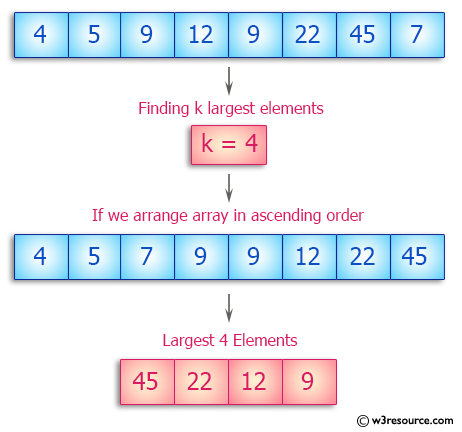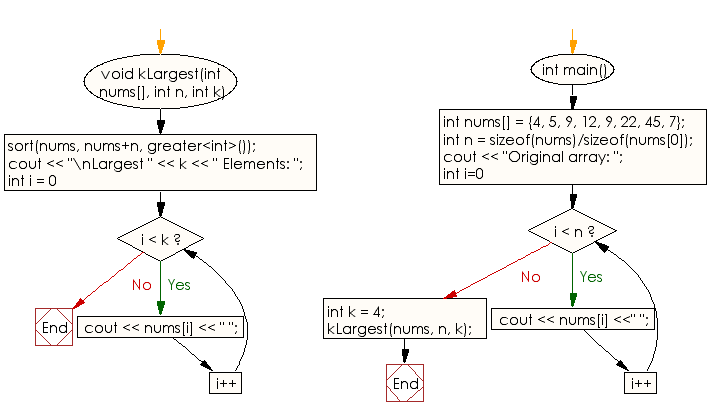﻿ C++ : Find k largest elements in a given array of integers# C++ Exercises: Find k largest elements in a given array of integers.

## C++ Array: Exercise-4 with Solution

Write a C++ program to find k largest elements in a given array of integers.

Pictorial Presentation:Sample Solution:

C++ Code :

``````#include<iostream>
using namespace std;
void kLargest(int nums[], int n, int k)
{
sort(nums, nums+n, greater<int>());
cout << "\nLargest " << k << " Elements: ";
for (int i = 0; i < k; i++)
cout << nums[i] << " ";
}

int main()
{
int nums[] = {4, 5, 9, 12, 9, 22, 45, 7};
int n = sizeof(nums)/sizeof(nums);
cout << "Original array: ";
for (int i=0; i < n; i++)
cout << nums[i] <<" ";
int k = 4;
kLargest(nums, n, k);
}
``````

Sample Output:

```Original array: 4 5 9 12 9 22 45 7
Largest 4 Elements: 45 22 12 9
```

Flowchart:C++ Code Editor:

What is the difficulty level of this exercise?

Test your Programming skills with w3resource's quiz.

﻿